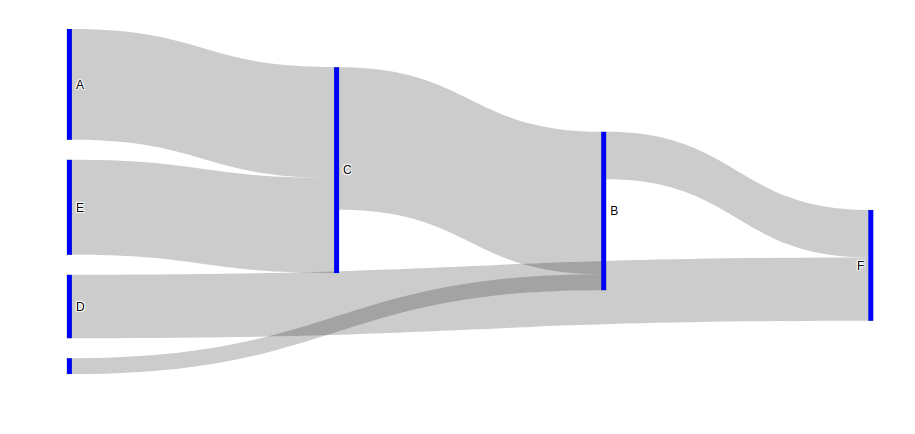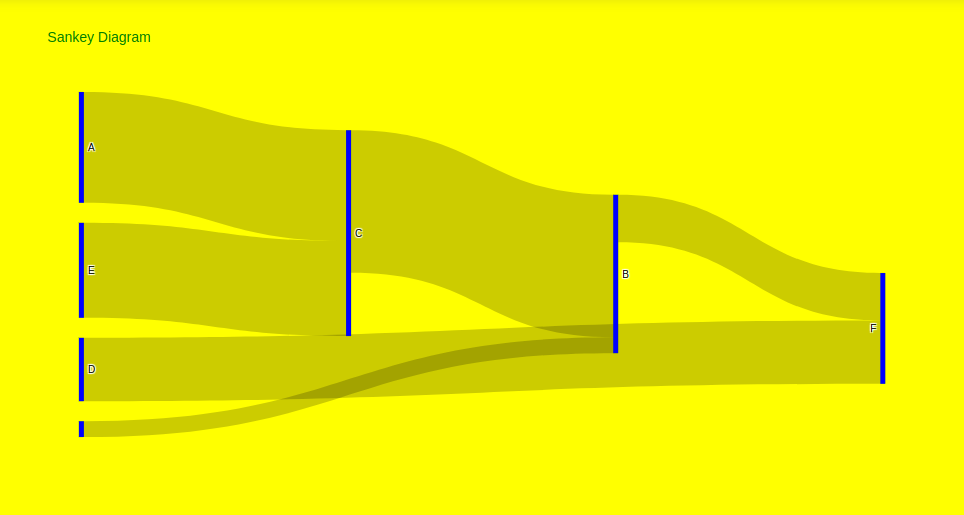Sankey Diagram using Plotly in Python

• Last Updated : 05 Sep, 2020

Plotly is a Python library that is used to design graphs, especially interactive graphs. It can plot various graphs and charts like histogram, barplot, boxplot, spreadplot, and many more. It is mainly used in data analysis as well as financial analysis. plotly is an interactive visualization library.

Sankey Diagrams

Sankey diagrams are a type of flow diagram in which the width of the arrows is comparative to the flow rate. Sankey diagrams can also visualize the source to represent the source node, target for the target node, value to set the flow volume and label that shows the node name. If the flow is twice as wide it represents double the quantity.

Example 1:

Python3

 fig = go.Figure(data=[go.Sankey(    node = dict(      thickness = 5,      line = dict(color = "green", width = 0.1),      label = ["A", "B", "C", "D", "E", "F"],      color = "blue"    ),    link = dict(                # indices correspond to labels      source = [0, 6, 1, 4, 2, 3],       target = [2, 1, 5, 2, 1, 5],      value = [7, 1, 3, 6, 9, 4]  ))])  fig.show()

Output:Example 2: Styling the graph

Python3

 fig = go.Figure(data=[go.Sankey(    node = dict(      thickness = 5,      line = dict(color = "green", width = 0.1),      label = ["A", "B", "C", "D", "E", "F"],      color = "blue"    ),    link = dict(                # indices correspond to labels      source = [0, 6, 1, 4, 2, 3],       target = [2, 1, 5, 2, 1, 5],      value = [7, 1, 3, 6, 9, 4]  ))])  fig.update_layout(    hovermode = 'x',    title="Sankey Diagram",    font=dict(size = 10, color = 'green'),    plot_bgcolor='blue',    paper_bgcolor='yellow')  fig.show()

Output:Attention geek! Strengthen your foundations with the Python Programming Foundation Course and learn the basics.

To begin with, your interview preparations Enhance your Data Structures concepts with the Python DS Course. And to begin with your Machine Learning Journey, join the Machine Learning - Basic Level Course

My Personal Notes arrow_drop_up